Related Topics: OpenGL Matrix, Quaternion to Matrix, Angles To Axes, Lookat To Axes

The 4x4 transformation matrix for rotating about an arbitrary axis in OpenGL is defined as;This page explains how to derive this rotation matrix using Rodrigues' formula. Suppose a 3D point P is rotating to Q by an anglealong a unit vector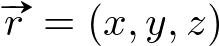. The vector form of P is broken up the sum of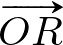and, and Q is the sum ofandrespectively;,Therefore, we need to findandfirst in order to get Q. The vectorcan be aquired from, andcomes from the circular plane, where the point Q lies on.is parallel to the unit vector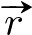, and its length can be computed by projectingtousing inner product. So, it can be written;Animation Of Rotating A Vertex About Arbitrary AxisRotating P to Q About Arbitrary Axiscan be determined by 2 basis vectors on the rotation plane. We useas the first basis vector, and the other basis vectoris perpendicular toand has equal length because they are both the radius of the circluar plane. Therefore,can be computed by the cross product of 2 perpendicular vectors;and;Now,is represented with the composition of these basis vectors and trigonometric functions;Finally, the rotated vector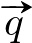is written by the sum ofand;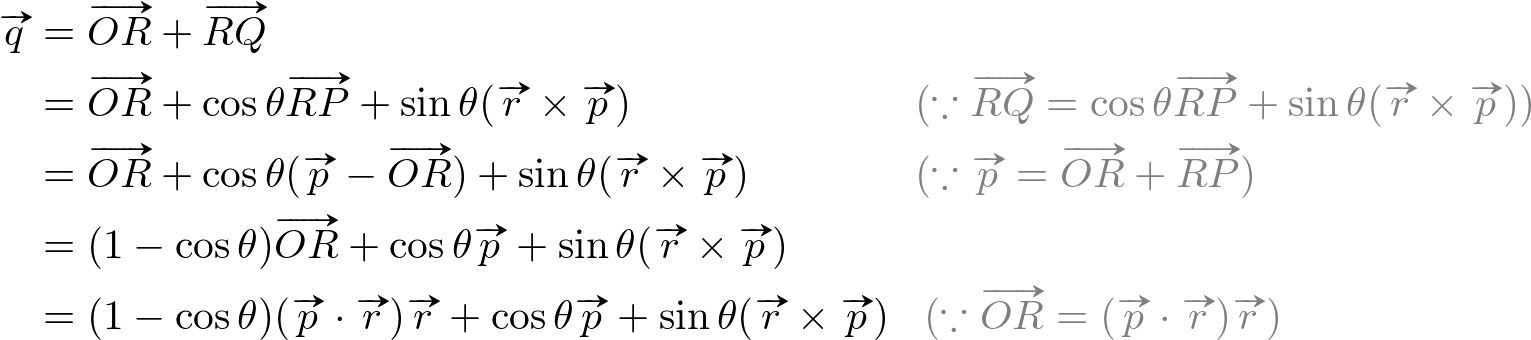This equation is called Rodrigues' rotation formula;It can be represented by an equivalent matrix form. First, convertandcomponents to 3x3 matrix forms for P = (px, py, pz) and r = (x, y, z);Finally, the equivalent matrix form by substituting the above matrix components is;And, the 3x3 rotation matrix alone is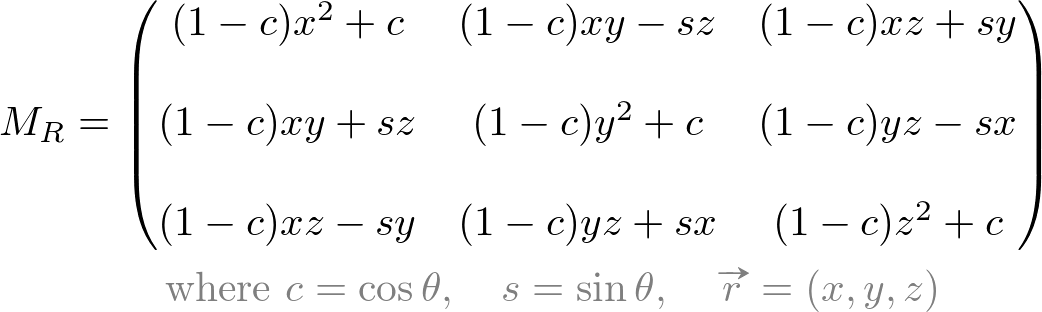Or, as 4x4 matrix;Example: Rodrigues' Rotation Formula

The following C++ code snippet is rotating a 3D point P to Q along the rotation axisusing Rodrigues' formula. Download the complete implementation from rotate.zip.// minimal implementation of Vector3
struct Vector3
{
float x, y, z;
// ctor
Vector3() : x(0), y(0), z(0) {}

// inner and cross products
float   dot(Vector3& v)   { return x*v.x + y*v.y + z*v.z; }
Vector3 cross(Vector3& v) { return Vector3(y*v.z-z*v.y, z*v.x-x*v.z, x*v.y-y*v.x); }
// scalar product
friend Vector3 operator*(float s, Vector3 v) { return Vector3(s*v.x, s*v.y, s*v.z); }
Vector3& normalize() {
float invLength = 1.0f / sqrtf(x*x + y*y + z*z);
x *= invLength;
y *= invLength;
z *= invLength;
return *this;
}
}
...

// define the rotation vector r and angle
Vector3 r = Vector3(1, 1, 1).normalize();   // make unit length
float a = 30 / 180 * PI;                    // rotation angle as radian

// define a vector p to rotate
Vector3 p = Vector3(1, 2, 3);

// compute the rotated vector q using Rodrigues' formula
Vector3 q = (1 - cos(a)) * p.dot(r) * r + cos(a) * p + sin(a) * r.cross(p);